# Winter 2023 Midterm Exam

Instructor(s): Suraj Rampure

This exam was administered in-person. The exam was closed-notes, except students were allowed to bring a single two-sided notes sheet. No calculators were allowed. Students had 50 minutes to take this exam.

Welcome to the Midterm Exam for DSC 80 in Winter 2023!

Throughout this exam, we will work with the DataFrame tv, which contains information about various TV shows available to watch on streaming services. For each TV show, we have:

• "Title" (object): The title of the TV show.
• "Year" (int): The year in which the TV show was first released. (For instance, the show How I Met Your Mother ran from 2005 to 2014; there is only one row for How I Met Your Mother in tv, and its "Year" value is 2005.)
• "Age" (object): The age category for the TV show. If not missing, "Age" is one of "all", "7+", "13+", "16+", or "18+". (For instance, "all" means that the show is appropriate for all audiences, while “18+”} means that the show contains mature content and viewers should be at least 18 years old.)
• "IMDb" (float): The TV show’s rating on IMDb (between 0 and 10).
• "Rotten Tomatoes" (int): The TV show’s rating on Rotten Tomatoes (between 0 and 100).
• "Netflix" (int): 1 if the show is available for streaming on Netflix and 0 otherwise. The "Hulu", "Prime Video", and "Disney+" columns work the same way.

The first few rows of tv are shown below (though tv has many more rows than are pictured here).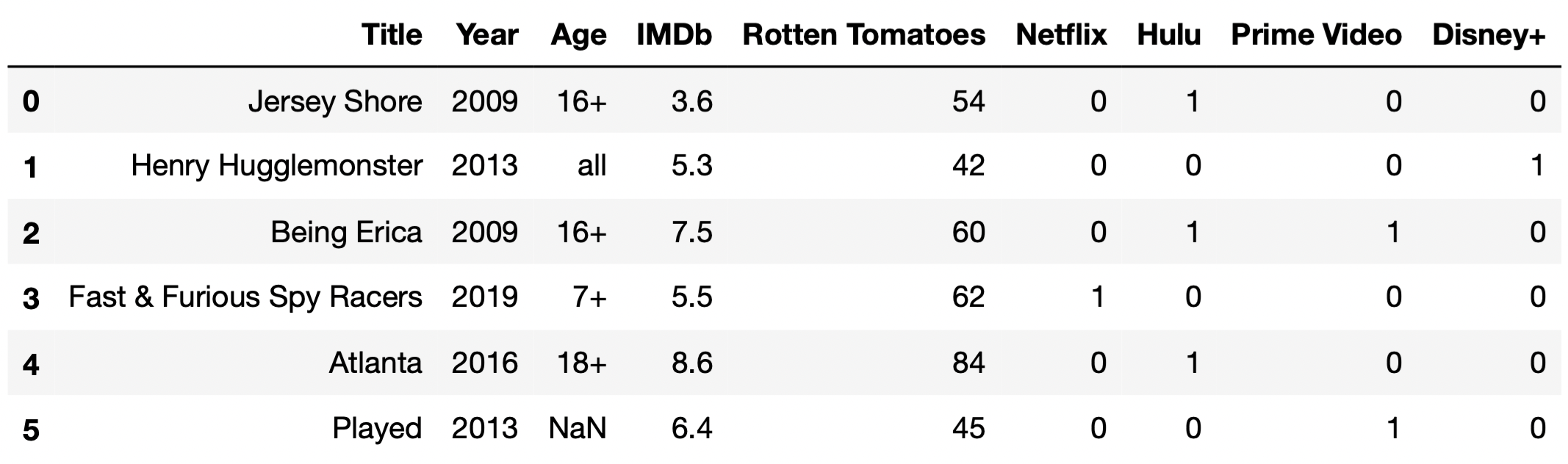Assume that we have already run all of the necessary imports.

Throughout this exam, we will refer to tv repeatedly.

## Problem 1

### Problem 1.1

What kind of variable is "Netflix"?

• Quantitative discrete

• Quantitative continuous

• Qualitative ordinal

• Qualitative nominal

Remember, a variable is quantitative (i.e. numerical) if arithmetic operations have meaning. If we add up the values in the "Netflix" column for a subset of the rows, we get the number of TV shows in that subset that are available for streaming on Netflix. If we take the average of the values in the "Netflix" column for a subset of the rows, we get the proportion of TV shows in that subset that are available for streaming on Netflix. Since arithmetic operations have meaning, "Netflix" is quantitative, and since it can only take on a finite number of values (just 0 or 1) it is also discrete.

##### Difficulty: ⭐️⭐️⭐️⭐️⭐️

The average score on this problem was 22%.

In the following subparts, consider the variable double_count, defined below.

double_count = tv["Title"].value_counts().value_counts()

### Problem 1.2

What is type(double_count)?

• Series

• SeriesGroupBy

• DataFrame

• DataFrameGroupBy

The .value_counts() method, when called on a Series s, produces a new Series in which

• the index contains all unique values in s.
• the values are the frequencies of the unique values in s.

Since tv["Title"] is a Series, tv["Title"].value_counts() is a Series, and so is tv["Title"].value_counts.value_counts(). We provide an interpretation of each of these Series in the solution to the next subpart.

##### Difficulty: ⭐️⭐️

The average score on this problem was 84%.

### Problem 1.3

Which of the following statements are true? Select all that apply.

• The only case in which it would make sense to set the index of tv to "Title" is if double_count.iloc == 1 is True.

• The only case in which it would make sense to set the index of tv to "Title" is if double_count.loc == tv.shape is True.

• If double_count.loc == 5 is True, there are 5 TV shows that all share the same "Title".

• If double_count.loc == 5 is True, there are 5 pairs of 2 TV shows such that each pair shares the same "Title".

• None of the above.

• The only case in which it would make sense to set the index of tv to "Title" is if double_count.loc == tv.shape is True.
• If double_count.loc == 5 is True, there are 5 pairs of 2 TV shows such that each pair shares the same "Title".

To answer, we need to understand what each of tv["Title"], tv["Title"].value_counts(), and tv["Title"].value_counts().value_counts() contain. To illustrate, let’s start with a basic, unrelated example. Suppose tv["Title"] looks like:

0    A
1    B
2    C
3    B
4    D
5    E
6    A
dtype: object

Then, tv["Title"].value_counts() looks like:

A    2
B    2
C    1
D    1
E    1
dtype: int64

and tv["Title"].value_counts().value_counts() looks like:

1    3
2    2
dtype: int64

Back to our actual dataset. tv["Title"], as we know, contains the name of each TV show. tv["Title"].value_counts() is a Series whose index is a sequence of the unique TV show titles in tv["Title"], and whose values are the frequencies of each title. tv["Title"].value_counts() may look something like the following:

Breaking Bad                             1
Fresh Meat                               1
Doctor Thorne                            1
...
Styling Hollywood                        1
Vai Anitta                               1
Fearless Adventures with Jack Randall    1
Name: Title, Length: 5368, dtype: int64

Then, tv["Title"].value_counts().value_counts() is a Series whose index is a sequence of the unique values in the above Series, and whose values are the frequencies of each value above. In the case where all titles in tv["Title"] are unique, then tv["Title"].value_counts() will only have one unique value, 1, repeated many times. Then, tv["Title"].value_counts().value_counts() will only have one row total, and will look something like:

1    5368
Name: Title, dtype: int64

This allows us to distinguish between the first two answer choices. The key is remembering that in order to set a column to the index, the column should only contain unique values, since the goal of the index is to provide a “name” (more formally, a label) for each row.

• The first answer choice, “The only case in which it would make sense to set the index of tv to "Title" is if double_count.iloc == 1 is True”, is false. As we can see in the example above, all titles are unique, but double_count.iloc is something other than 1.
• The second answer choice, “The only case in which it would make sense to set the index of tv to "Title" is if double_count.loc == tv.shape is True”, is true. If double_count.loc == tv.shape, it means that all values in tv["Title"].value_counts() were 1, meaning that tv["Title"] consisted solely of unique values, which is the only case in which it makes sense to set "Title" to the index.

Now, let’s look at the second two answer choices. If double_counts.loc == 5, it would mean that 5 of the values in tv["Title"].value_counts() were 2. This would mean that there were 5 pairs of titles in tv["Title"] that were the same.

• This makes the fourth answer choice, “If double_count.loc == 5 is True, there are 5 pairs of 2 TV shows such that each pair shares the same "Title"”, correct.
• The third answer choice, “If double_count.loc == 5 is True, there are 5 TV shows that all share the same "Title"”, is incorrect; if there were 5 TV shows with the same title, then double_count.loc would be at least 1, but we can’t make any guarantees about double_counts.loc.

##### Difficulty: ⭐️⭐️⭐️

The average score on this problem was 68%.

## Problem 2

Ethan is an avid Star Wars fan, and the only streaming service he has an account on is Disney+. (He had a Netflix account, but then Netflix cracked down on password sharing.)

Fill in the blanks below so that star_disney_prop evaluates to the proportion of TV shows in tv with "Star Wars" in the title that are available to stream on Disney+.

star_only = __(a)__
star_disney_prop = __(b)__ / star_only.shape

What goes in the blanks?

• Blank (a): tv[tv["Title"].str.contains("Star Wars")]
• Blank (b): star_only["Disney+"].sum()

We’re asked to find the proportion of TV shows with "Star Wars" in the title that are available to stream on Disney+. This is a fraction, where:

• The numerator is the number of TV shows that have "Star Wars" in the title and are available to stream on Disney+.
• The denominator is the number of TV shows that have "Star Wars" in the title.

The key is recognizing that star_only must be a DataFrame that contains all the rows in which the "Title" contains "Star Wars"; to create this DataFrame in blank (a), we use tv[tv["Title"].str.contains("Star Wars")]. Then, the denominator is already provided for us, and all we need to fill in is the numerator. There are a few possibilities, though they all include star_only:

• star_only["Disney+"].sum()
• (star_only["Disney+"] == 1).sum()
• star_only[star_only["Disney+"] == 1].shape

Common misconception: Many students calculated the wrong proportion: they calculated the proportion of shows available to stream on Disney+ that have "Star Wars" in the title. We asked for the proportion of shows with "Star Wars" in the title that are available to stream on Disney+; “proportion of X that Y” is always \frac{\# X \text{ and } Y}{\# X}.

##### Difficulty: ⭐️⭐️

The average score on this problem was 84%.

## Problem 3

As you saw in the first few rows of tv, some TV shows are available for streaming on multiple streaming services. Fill in the blanks so that the two expressions below, Expression 1 and Expression 2, both evaluate to the "Title" of the TV show that is available for streaming on the greatest number of streaming services. Assume there are no ties and that the "Title" column contains unique values.

Expression 1:

tv.set_index("Title").loc[__(a)__].T.sum(axis=0).idxmax()

Expression 2:

    (
tv.assign(num_services=tv.iloc[__(b)__].sum(__(c)__))
.sort_values("num_services")
.iloc[__(d)__]
)

Hint: .T transposes the rows and columns of a DataFrame — the indexes of df are the columns of df.T and vice versa.

What goes in the blanks?

1. :, "Netflix": or some variation of that
1. :, 5: or some variation of that
1. axis=1
1. -1, 0

In Expression 1, keep in mind that idxmax() is a Series method returns the index of the row with the maximum value. As such, we can infer that Expression 1 sums the service-specific indicator columns (that is, the columns "Netflix", "Hulu", "Prime Video", and "Disney+") for each row and returns the index of the row with the greatest sum. To do this, we need the loc accessor to select all the service-specific indicator columns, which we can do using loc[:, "Netflix":] or loc[:, ["Netflix", "Hulu", "Prime Video", "Disney+"]].

When looking at Expression 2, we can split the problem into two parts: the code inside the assign statement and the code outside of it.

• Glancing at the code inside of the assign statement, (and also noticing the variable num_services), we realize that we, once again, want to sum up the values in the service-specific indicator columns. We do this by first selecting the last four columns, using .iloc[:, 5:] (notice the iloc), and then summing over axis=1. We use axis=1 (different from axis=0 in Expression 1), because unlike Expression 1, we’re summing over each row, instead of each column. If there had not been a .T in the code for Expression 1, we would’ve also used axis=1 in Expression 1.
• Finally, we need to select the "Title" of the last row in DataFrame in Expression 2, because sort_values sorts in ascending order by default. The last row has an integer position of -1, and the "Title" column has an integer position of 0, so we use iloc[-1, 0].

##### Difficulty: ⭐️⭐️⭐️

The average score on this problem was 69%.

## Problem 4

In 2020, during the early COVID era, popular shows like Never Have I Ever, The Last Dance, and The Queen’s Gambit were all released. What they all share in common, other than high viewership numbers and popularity on social media, are high ratings on both IMDb and Rotten Tomatoes.

Fill in the blank below so that top_years evaluates to an array of the years in which at least 5 TV shows with an IMDb rating of 9 or higher were released.

top_years = tv.groupby("Year").filter(____)["Year"].unique()

What goes in the blank?

Answer: lambda df: (df["IMDb"] >= 9).sum() >= 5

The filter method of a DataFrameGroupBy object takes in a function. That function should itself take in a DataFrame, corresponding to all of the rows for a particular "Year", and return either True or False. The result, tv.groupby("Year").filter(<our function>), will be a DataFrame containing only the rows in which the returned Boolean by our function is True. For instance, tv.groupby("Year").filter(lambda df: df.shape >= 2) will contain all of the rows for "Years" with at least 2 TV shows.

In our case, we want tv.groupby("Year").filter(<our function>) to evaluate to a DataFrame with all of the "Years" that have at least 5 TV shows that have an "IMDb" rating of at least 9 (since the provided code afterwards, ["Year"].unique(), finds all of the unique "Year"s in the DataFrame we produce). If df is a DataFrame of TV shows, then (df["IMDb"] >= 9).sum() is the number of TV shows in that DataFrame with an "IMDb" rating of at least 9, and (df["IMDb"] >= 9).sum() >= 5 is True only for DataFrames in which there are at least 5 TV shows with an "IMDb" rating of at least 9. Thus, the answer we were looking for is lambda df: (df["IMDb"] >= 9).sum() >= 5.

Another good answer we saw was lambda df: df.loc[df["IMDb"] >= 9, "Title"].nunique() >= 5.

Fun fact: In the DataFrame we used to produce the exam, the only year that satisfied the above criteria was 2020!

##### Difficulty: ⭐️⭐️⭐️

The average score on this problem was 56%.

## Problem 5

For your convenience, the first few rows of tv are shown again below.For the purposes of this question only, suppose we have also access to another similar DataFrame, movies, which contains information about a variety of movies. The information we have for each movie in movies is the same as the information we have for each TV show in tv, except for IMDb ratings, which are missing from movies.

The first few rows of movies are shown below (though movies has many more rows than are pictured here).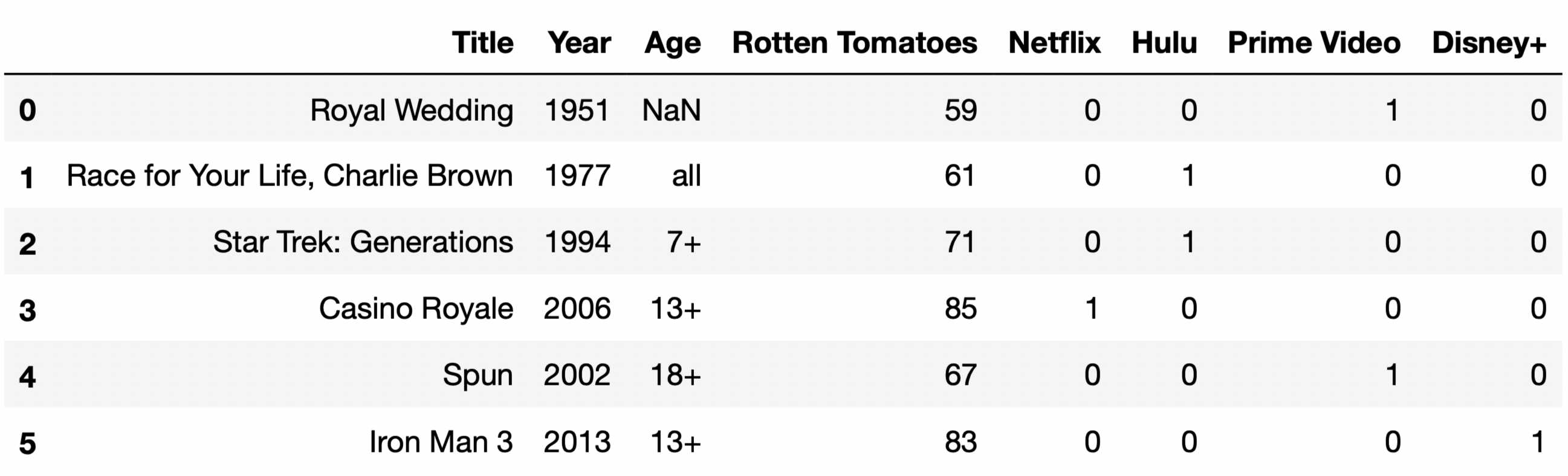### Problem 5.1

The function total_null, defined below, takes in a DataFrame and returns the total number of null values in the DataFrame.

total_null = lambda df: df.isna().sum().sum()

Consider the function delta, defined below.

def delta(a, b):
together = pd.concat([tv_a, movies_b])
return total_null(together) - total_null(tv_a) - total_null(movies_b)

Which of the following functions is equivalent to delta?

• lambda a, b: a

• lambda a, b: b

• lambda a, b: 9 * a

• lambda a, b: 8 * b

• lambda a, b: min(9 * a, 8 * b)

Answer: lambda a, b: b

Let’s understand what each function does.

• total_null just counts all the null values in a DataFrame.
• delta concatenates the first a rows of tv with the first b rows of movies vertically, that is, on top of one another (over axis 0). It then returns the difference between the total number of null values in the concatenated DataFrame and the total number of null values in the first a rows of tv and first b rows of movies – in other words, it returns the number of null values that were added as a result of the concatenation.

The key here is recognizing that tv and movies have all of the same column names, except movies doesn’t have an "IMDb" column. As a result, when we concatenate, the "IMDb" column will contain null values for every row that was originally from movies. Since b rows from movies are in the concatenated DataFrame, b new null values are introduced as a result of the concatenation, and thus lambda, a, b: b does the same thing as delta.

##### Difficulty: ⭐️⭐️⭐️

The average score on this problem was 58%.

### Problem 5.2

Fill in the blank to complete the implementation of the function size_of_merge, which takes a string col, corresponding to the name of a single column that is shared between tv and movies, and returns the number of rows in the DataFrame tv.merge(movies, on=col).

• For instance, size_of_merge("Year") should return the number of rows in tv.merge(movies, on="Year").

• The purpose of this question is to have you think conceptually about how merges work. As such, solutions containing merge or concat will receive 0 points.

What goes in the blank below?

def size_of_merge(col):
return (____).sum()

Hint: Consider the behavior below.

>>> s1 = pd.Series({'a': 2, 'b': 3})
>>> s2 = pd.Series({'c': 4, 'a': -1, 'b': 4})
>>> s1 * s2
a    -2.0
b    12.0
c     NaN
dtype: float64

Answer: tv[col].value_counts() * movies[col].value_counts()

tv.merge(movies, on=col) contains one row for every “match” between tv[col] and movies[col]. Suppose, for example, that col="Year". If tv["Year"] contains 30 values equal to 2019, and movies["Year"] contains 5 values equal to 2019, tv.merge(movies, on="Year") will contain 30 \cdot 5 = 150 rows in which the "Year" value is equal to 2019 – one for every combination of a 2019 row in tv and a 2019 row in movies.

tv["Year"].value_counts() and movies["Year"].value_counts() contain, respectively, the frequencies of the unique values in tv["Year"] and movies["Year"]. Using the 2019 example from above, tv["Year"].value_counts() * movies["Year"].value_counts() will contain a row whose index is 2019 and whose value is 150, with similar other entries for the other years in the two Series. (The hint is meant to demonstrate the fact that no matter how the two Series are sorted, the product is done element-wise by matching up indexes.) Then, (tv["Year"].value_counts() * movies["Year"].value_counts()).sum() will sum these products across all years, ignoring null values.

As such, the answer we were looking for is tv[col].value_counts() * movies[col].value_counts() (remember, "Year" was just an example for this explanation).

##### Difficulty: ⭐️⭐️⭐️⭐️⭐️

The average score on this problem was 14%.

## Problem 6

For the remainder of the exam, we will only consider TV shows that are available to stream exclusively on a single streaming service. The DataFrame tv_excl contains all of the information we have for these TV shows. Note that instead of containing separate columns for each streaming service, as tv did, tv_excl instead has a single "Service" column that contains the name of the one streaming service that the TV show is available for streaming on.

The first few rows of tv_excl are shown below (though, of course, tv_excl has many more rows than are pictured here). Note that Being Erica is not in tv_excl, since it is available to stream on multiple services.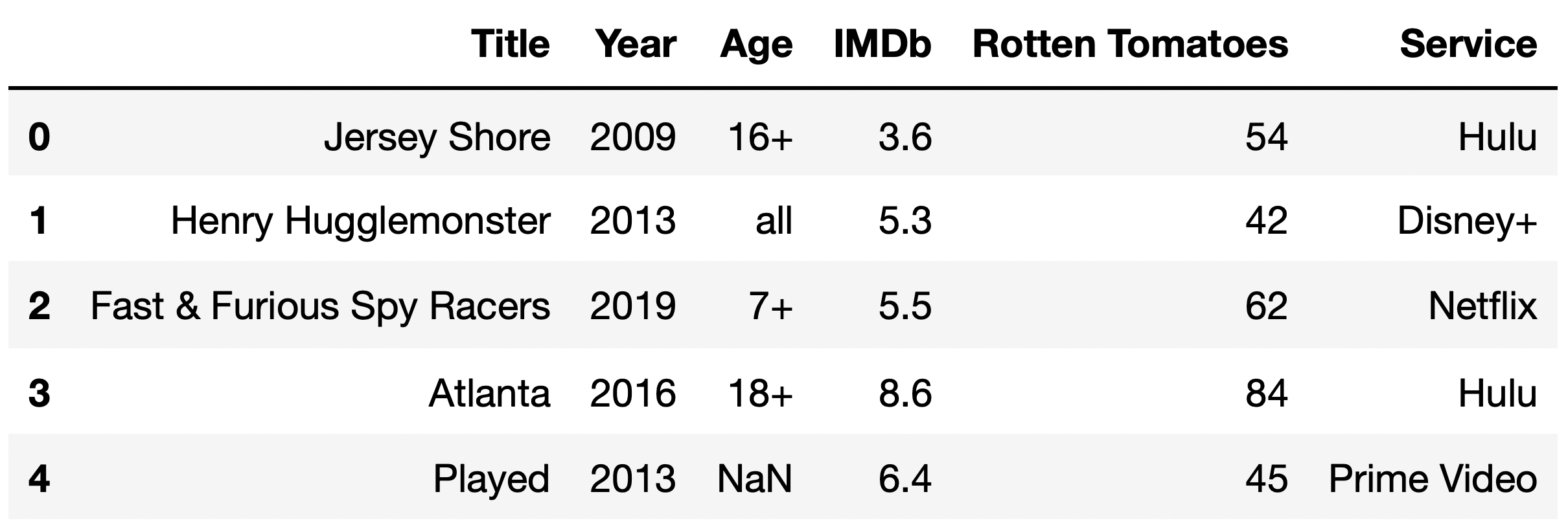### Problem 6.1

The DataFrame counts, shown in full below, contains the number of TV shows for every combination of "Age" and "Service".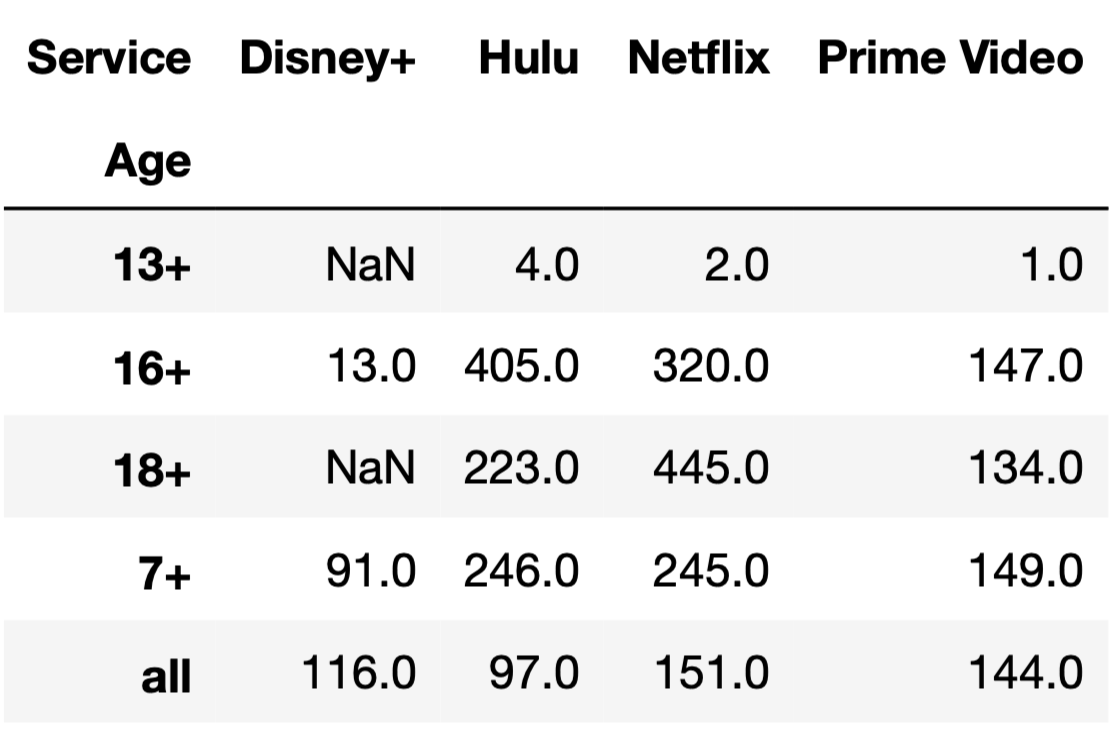Given the above information, what does the following expression evaluate to?

tv_excl.groupby(["Age", "Service"]).sum().shape
• 4

• 5

• 12

• 16

• 18

• 20

• 25

Note that the DataFrame counts is a pivot table, created using tv_excl.pivot_table(index="Age", columns="Service", aggfunc="size"). As we saw in lecture, pivot tables contain the same information as the result of grouping on two columns.

The DataFrame tv_excl.groupby(["Age", "Service"]).sum() will have one row for every unique combination of "Age" and "Service" in tv_excl. (The same is true even if we used a different aggregation method, like .mean() or .max().) As counts shows us, tv_excl contains every possible combination of a single element in {"13+", "16+", "18+", "7+", "all"} with a single element in {"Disney+", "Hulu", "Netflix", "Prime Video"}, except for ("13+", "Disney+") and ("18+", "Disney+"), which were not present in tv_excl; if they were, they would have non-null values in counts.

As such, tv_excl.groupby(["Age", "Service"]).sum() will have 20 - 2 = 18 rows, and tv_excl.groupby(["Age", "Service"]).sum().shape evaluates to 18.

##### Difficulty: ⭐️⭐️⭐️⭐️

The average score on this problem was 34%.

### Problem 6.2

Tiffany would like to compare the distribution of "Age" for Hulu and Netflix. Specifically, she’d like to test the following hypotheses:

• Null Hypothesis: The distributions of "Age" for Hulu and Netflix are drawn from the same population distribution, and any observed differences are due to random chance.

• Alternative Hypothesis: The distributions of "Age" for Hulu and Netflix are drawn from different population distributions.

What type of test is this?

• Hypothesis test

• Permutation test

A permutation test is a statistical test in which we aim to determine if two samples look like they were drawn from the same unknown population. Here, our two samples are the distribution of "Age"s for Hulu and the distribution of "Age"s for Netflix.

##### Difficulty: ⭐️

The average score on this problem was 97%.

### Problem 6.3

Consider the DataFrame distr, defined below.

hn = counts[["Hulu", "Netflix"]]
distr = (hn / hn.sum()).T  # Note that distr has 2 rows and 5 columns.

To test the hypotheses in part (b), Tiffany decides to use the total variation distance as her test statistic. Which of the following expressions DO NOT correctly compute the observed statistic for her test? Select all that apply.

• distr.diff().iloc[-1].abs().sum() / 2

• distr.diff().sum().abs().sum() / 2

• distr.diff().sum().sum().abs() / 2

• (distr.sum() - 2 * distr.iloc).abs().sum() / 2

• distr.diff().abs().sum(axis=1).iloc[-1] / 2

• None of the above: all above options correctly compute the observed statistic.

Answer: distr.diff().sum().sum().abs() / 2 only

First, note that the difference between the TVD calculation here and those in lecture is that our pivot table contains one row for each distribution, rather than one column for each distribution. This is because of the .T in the code snippet above. distr may look something like: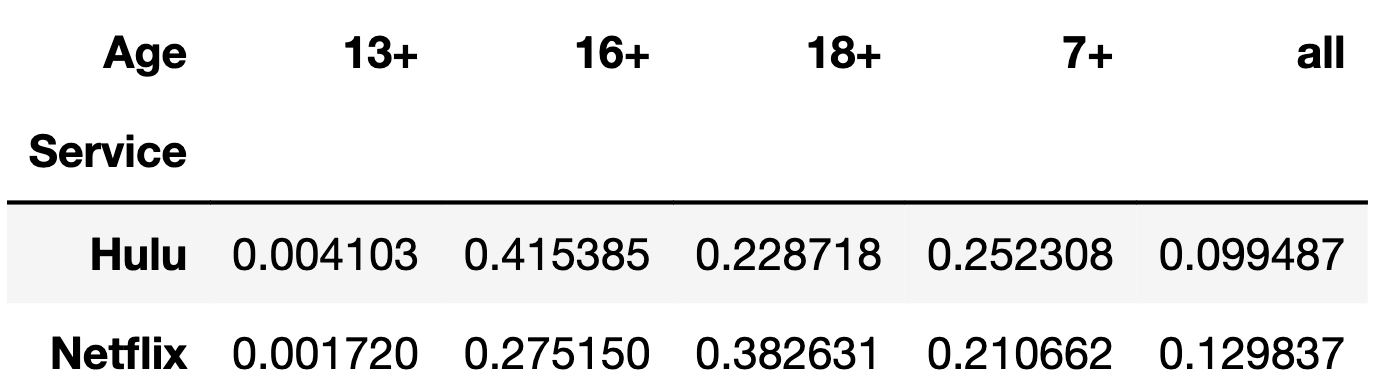As such, here we need to apply the .diff() method to each column first, not each row (meaning we should supply axis=0 to diff, not axis=1; axis=0 is the default, so we don’t need to explicitly specify it). distr.diff() may look something like: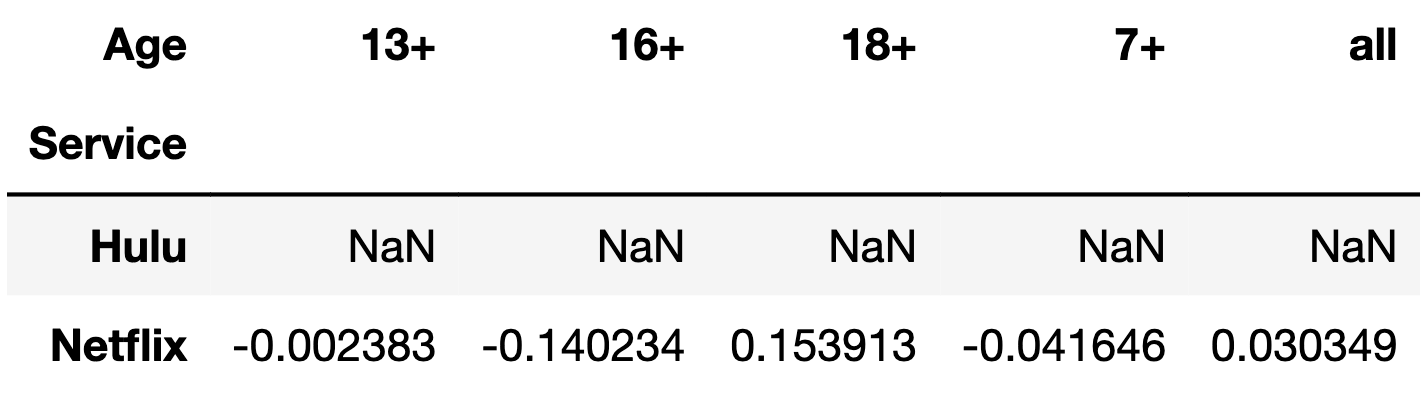With that in mind, let’s look at each option, remembering that the TVD is the sum of the absolute differences in proportions, divided by 2.

• distr.diff().iloc[-1].abs().sum() / 2:
• distr.diff().iloc[-1] contains the differences in proportions.
• distr.diff().iloc[-1].abs() contains the absolute differences in proportions.
• distr.diff().iloc[-1].abs().sum() / 2 contains the sum of the absolute differences in proportions, divided by 2. This is the TVD.
• distr.diff().sum().abs().sum() / 2:
• distr.diff().sum() is a Series containing just the last row in distr.diff(); remember, null values are ignored when using methods such as .mean() and .sum().
• distr.diff().sum().abs() contains the absolute differences in proportions, and hence distr.diff().sum().abs().sum() / 2 contains the sum of the absolute differences in proportions, divided by 2. This is the TVD.
• distr.diff().sum().sum().abs() / 2:
• distr.diff().sum() contains the differences in proportions (explained above).
• distr.diff().sum().sum() contains the sum of the differences in proportions. This is 0; remember, the reason we use the absolute value is to prevent the positive and negative differences in proportions from cancelling each other out. As a result, this option does not compute the TVD; in fact, it errors, because distr.diff().sum().sum() is a single float, and floats don’t have an .abs() method.
• (distr.sum() - 2 * distr.iloc).abs().sum() / 2:
• This option seems strange, but does actually compute the TVD. The key idea is the fact that a - b is the same as (a + b) - 2 \cdot b). distr.sum() is the same as distr.iloc + distr.iloc, so distr.sum() - 2 * distr.iloc is distr.iloc + distr.iloc - 2 * distr.iloc which is distr.iloc - distr.iloc, which is just distr.diff().iloc[-1].
• Then, this option reduces to distr.diff().iloc[-1].abs().sum() / 2, which is the same as Option 1. This is the TVD.
• distr.diff().abs().sum(axis=1).iloc[-1] / 2:
• distr.diff().abs() is a DataFrame in which the last row contains the absolute differences in proportions.
• distr.diff().abs().sum(axis=1) is a Series in which the first element is null and the second element is the sum of the absolute differences in proportions.
• As such, distr.diff().abs().sum(axis=1).iloc[-1] / 2 is the sum of the absolute differences in proportions divided by 2. This is the TVD.

##### Difficulty: ⭐️⭐️⭐️

The average score on this problem was 67%.

Doris proposes a novel approach for testing the hypotheses in part (b). She proposes we compute the distribution of "Age" for all TV shows in tv_excl, ignoring the streaming service they’re available on. Then, she suggests we run two separate tests of the following hypotheses, using the same significance level as used for the tests in part (b):

• Null Hypothesis: The distribution of "Age" for service X is drawn from the distribution of "Age" for all services we have data for.

• Alternative Hypothesis: The distribution of "Age" for service X is not drawn from the distribution of "Age" for all services we have data for.

She suggests we test the above pair of hypotheses separately for Hulu and Netflix, and gives the following interpretations:

1. If we fail to reject both null hypotheses here, we can also fail to reject the null hypothesis in part (b).

2. If we reject both null hypotheses here, we can also reject the null hypothesis in part (b).

### Problem 6.4

What type of test is Doris proposing we run?

• Hypothesis test

• Permutation test

A hypothesis test is a statistical test in which we aim to determine whether a sample looks like it was drawn at random from a known population. Here, Doris is proposing we run two separate hypothesis tests: one in which we determine whether the distribution of "Age" for Hulu (sample) is drawn from the distribution of "Age" in our entire dataset (population), and one in which we determine whether the distribution of "Age" for Netflix (sample) is drawn from the distribution of "Age" in our entire dataset (population).

##### Difficulty: ⭐️

The average score on this problem was 93%.

### Problem 6.5

Which of Doris’ interpretations are valid? Select all that apply.

• Interpretation 1: If we fail to reject both null hypotheses here, we can also fail to reject the null hypothesis in Problem 6.2.

• Interpretation 2: If we reject both null hypotheses here, we can also reject the null hypothesis in Problem 6.2.

• Neither interpretation is valid.

Let’s consider each option.

• Interpretation 1: Suppose we fail to reject both null hypotheses here. If that’s the case, then the distribution of "Age" for Hulu looks like a random sample from the distribution of "Age" in our full dataset, and so does the distribution of "Age" for Netflix. (Note that we can’t conclude they are random samples from the distribution of "Age" in our full dataset, since we can’t prove the null, we can only fail to reject it). If that’s the case, the distributions of "Age" for Hulu and Netflix both look like they’re drawn from the same population, which means we fail to reject the null from Problem 6.2.
• Interpretation 2: Suppose we reject both null hypotheses here. If that’s the case, then neither the distribution of "Age" for Hulu nor the distribution of "Age" for Netflix look like a random sample of "Age" in our full dataset. However, that doesn’t imply that these two distributions don’t look like samples of the same population; all it implies is that they don’t look like samples of this particular population. It is still possible that there exists some population distribution that the distributions of "Age" for Hulu and Netflix both look like they’re drawn from, which means we can’t automatically reject the null from Problem 6.2.

##### Difficulty: ⭐️⭐️⭐️

The average score on this problem was 60%.

## Problem 7

The "IMDb" column in tv_excl contains several missing values.

Note: Answer each subpart of this question independently of other subparts.

### Problem 7.1

Given no other information other than a TV show’s "Title" and "IMDb" rating, what is the most likely missingness mechanism of the "IMDb" column?

• Missing by design

• Not missing at random

• Missing at random

• Missing completely at random

• Full credit: Not missing at random
• Partial credit: Missing completely at random

The answer we were looking for is not missing at random (NMAR). As we saw repeatedly in lectures and Lab 5, in cases where all we have access to is a single column with missing values, potentially with other unrelated columns (like "Title" here), the best explanation is that there is some inherent reason as to why the values in the column with missing values are missing. Here, a reasonable interpretation is that the "IMDb" scores that are missing are likely to come from worse TV shows, and so lower scores are more likely to be missing. Think about it like this – if a TV show is really great, presumably more people would know about it, and it would be rated. If a TV show wasn’t as good and wasn’t as popular, it is more likely to be ignored.

However, partial credit was awarded to those who answered missing completely at random.

##### Difficulty: ⭐️⭐️⭐️

The average score on this problem was 63%.

### Problem 7.2

Now, suppose we discover that the median "Rotten Tomatoes" rating among TV shows with a missing "IMDb" rating is a 13, while the median "Rotten Tomatoes" rating among TV shows with a present "IMDb" rating is a 52.

Given this information, what is the most likely missingness mechanism of the "IMDb" column?

• Missing by design

• Not missing at random

• Missing at random

• Missing completely at random

The problem tells us that the distribution of "Rotten Tomatoes" when "IMDb" is missing (mean 13) is very different from the distribution of "Rotten Tomatoes" when "IMDb" is not missing (mean 52). As such, the missingness of "IMDb" appears to depend on "Rotten Tomatoes", and so the most likely missingness mechanism is missing at random.

##### Difficulty: ⭐️⭐️

The average score on this problem was 83%.

### Problem 7.3

Suppose we want to perform a statistical test to determine whether the missingness of "IMDb" depends on "Age". Which of the following test statistics could we use? Select all that apply.

• Difference in means

• Absolute difference in means

• Total variation distance

• The Kolmogorov-Smirnov statistic

• None of the above

Our permutation test here needs to compare two distributions:

• The distribution of "Age" when "IMDb" is missing.
• The distribution of "Age" when "IMDb" is not missing.

Since "Age" is a categorical variable – remember, its only possible values are "7+", "13+", "16+", "18+", and "all" – the above two distributions are categorical. The only test statistic of the options provided that compares categorical distributions is the total variation distance.

##### Difficulty: ⭐️⭐️⭐️

The average score on this problem was 63%.

### Problem 7.4

To determine whether the missingness of "IMDb" depends on "Year", we produce the following plot.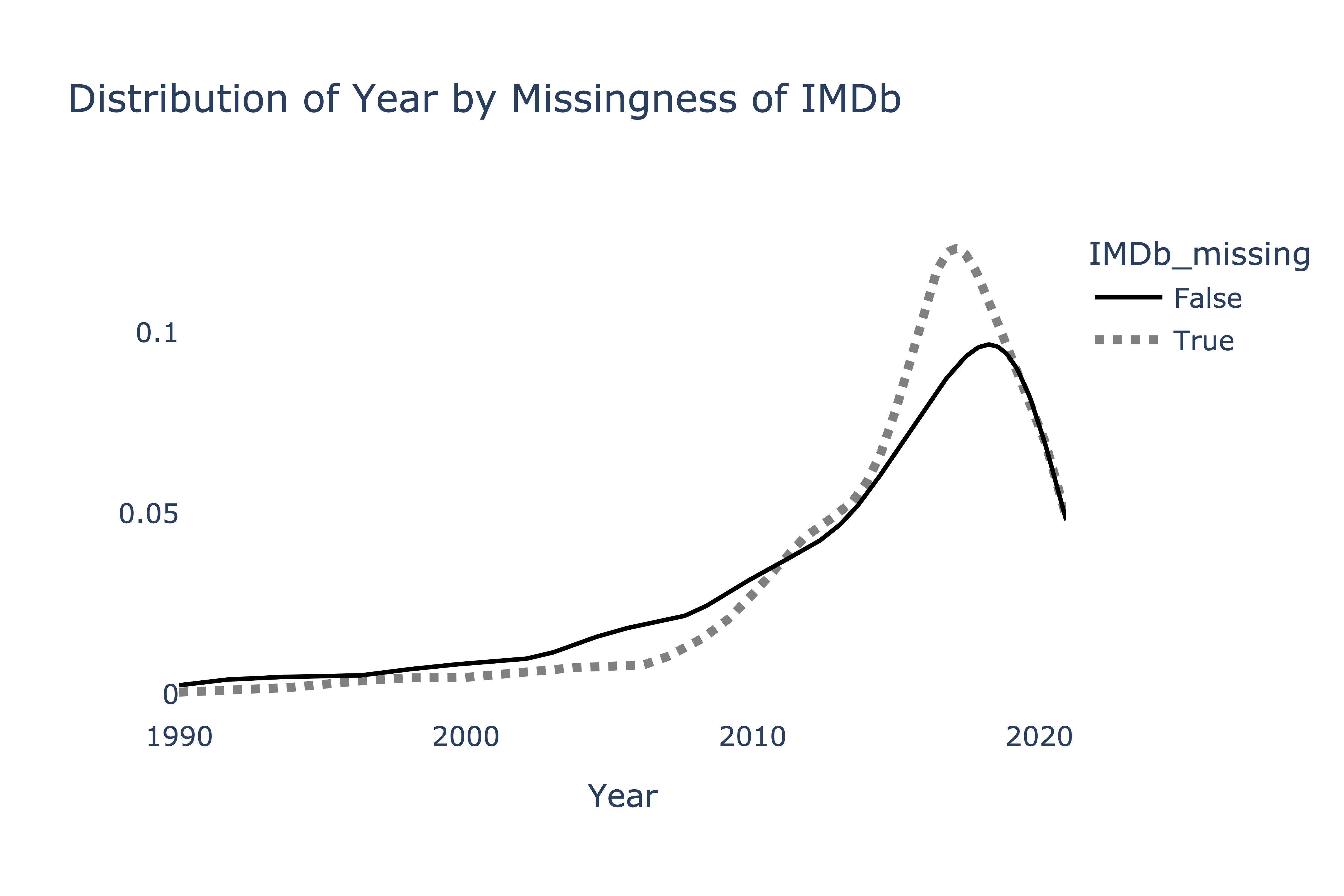Suppose we want to perform a statistical test to determine whether the two distributions above come from the same population distribution. Which test statistic is most likely to yield a significant result?

• Difference in means

• Absolute difference in means

• Total variation distance

• The Kolmogorov-Smirnov statistic

First, note that the two distributions are quantitative, which means the TVD can’t be used here (the TVD only measures the difference between two categorical distributions).

To decide between the remaining options, note that the two distributions visualized appear to have the same mean, but different shapes. The Kolmogorov-Smirnov statistic is designed to detect differences in the shapes of distributions with the same center, and as such, it is the most likely to yield a significant result here. The others may not; since the means of the two distributions are very similar, the observed difference in means will be close to 0, which is a typical value under the null.

##### Difficulty: ⭐️⭐️

The average score on this problem was 89%.

### Problem 7.5

To determine whether the missingness of "IMDb" depends on "Service", we produce the following plot.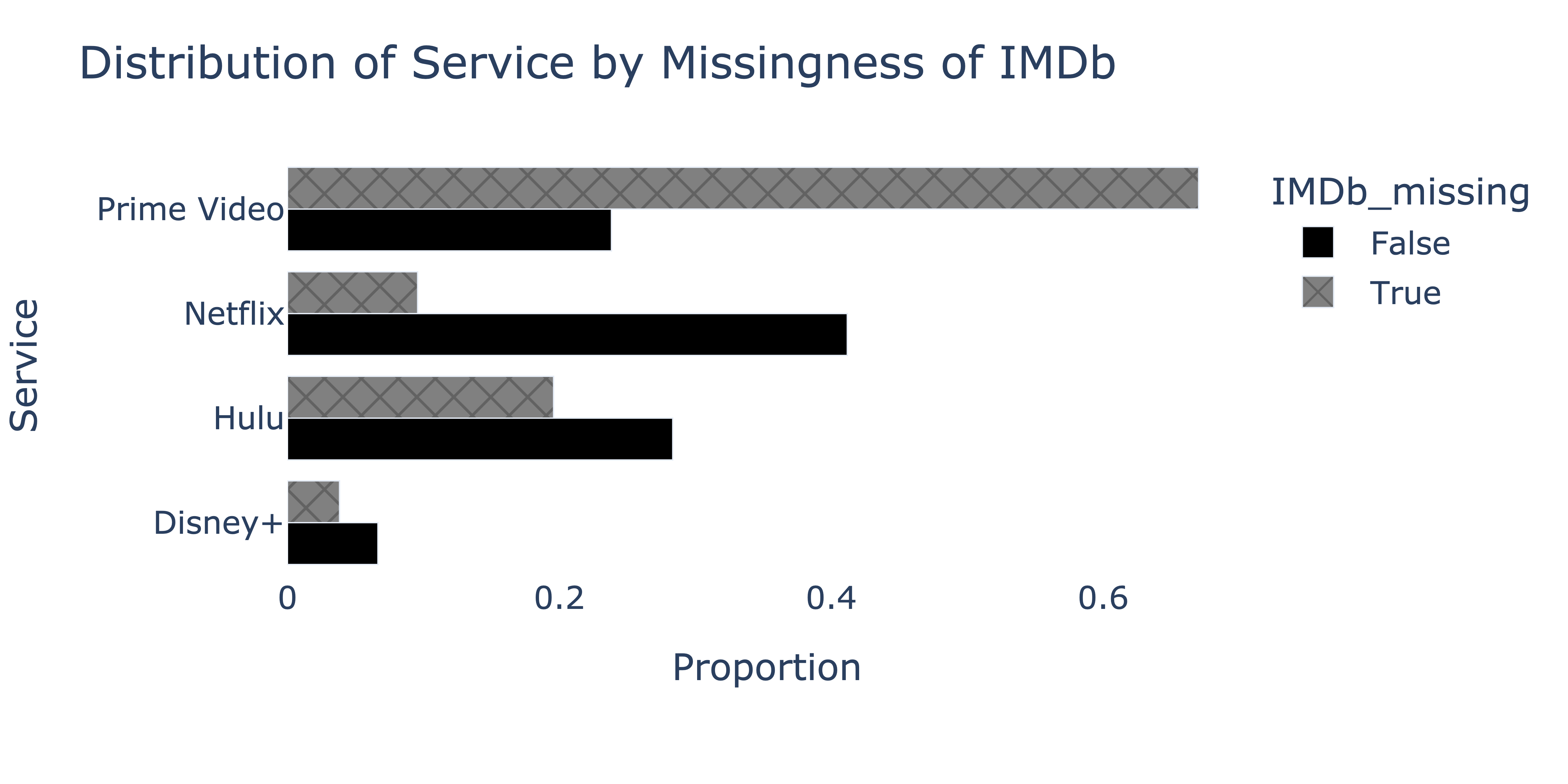We’d like to fill in missing "IMDb" values in the fastest, most efficient way possible, such that the mean of the imputed "IMDb" column is as close to the true mean of the "IMDb" column in nature as possible. Which imputation technique should we use?

• Unconditional mean imputation

• Mean imputation, conditional on "Service"

• Unconditional probabilistic imputation

• Probabilistic imputation, conditional on "Service"

Since the missingness of "IMDb" appears to depend on "Service", in order to accurately estimate the true mean of the "IMDb" column, we must impute conditionally on "Service"`, otherwise the imputed mean will be biased.

To decide between conditional mean imputation and conditional probabilistic imputation, note that we were asked to find the fasted, most efficient technique possible, such that the imputed mean is close to the true mean. Conditional mean imputation is more efficient than conditional probabilistic imputation, as probabilistic imputation requires sampling. While mean imputation shrinks the variance of the imputed distribution relative to the true distribution, we weren’t asked to preserve the variance of the true distribution, so conditional mean imputation is the right choice.

##### Difficulty: ⭐️⭐️⭐️⭐️

The average score on this problem was 46%.